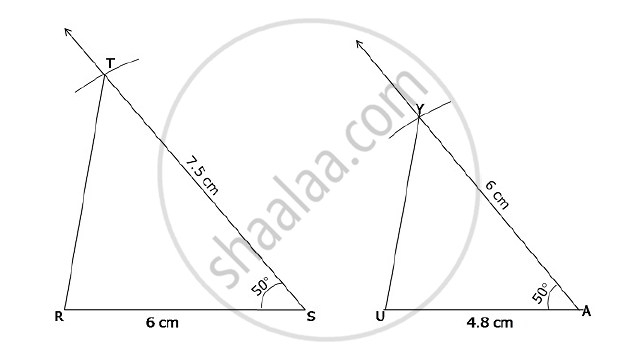# ΔRST ~ ΔUAY, In ΔRST, RS = 6 cm, ∠S = 50°, ST = 7.5 cm. The corresponding sides of ΔRST and ΔUAY are in the ratio 5 : 4. Construct ΔUAY. - Geometry

ΔRST ~ ΔUAY, In ΔRST, RS = 6 cm, ∠S = 50°, ST = 7.5 cm. The corresponding sides of ΔRST and ΔUAY are in the ratio 5 : 4. Construct ΔUAY.

#### Solution

Given that ΔRST ~ ΔUAY.

In ΔRST, RS = 6 cm, m∠S = 50°, ST = 7.5 cm.

Given that the corresponding sides of ΔRST and ΔUAY are in the ratio 5 : 4.

therefore "RS"/"UA"="ST"/"AY"="RT"/"UY"=5/4;

∠S =∠A = 50º

therefore"RS"/"UA"=5/4

therefore6/"UA"=5/4

therefore(6xx4)/5="UA"

therefore "UA"=4.8 "cm"

Similarly,

"ST"/"AY"=5/4;

therefore7.5/"AY"=5/4

therefore(7.5xx4)/5="AY"

therefore"AY"=6" cm"

Therefore, In ΔUAY, UA = 4.8 cm, AY = 6 cm and m∠A = 50°Concept: Division of a Line Segment
Is there an error in this question or solution?
2014-2015 (March) Set B

Share